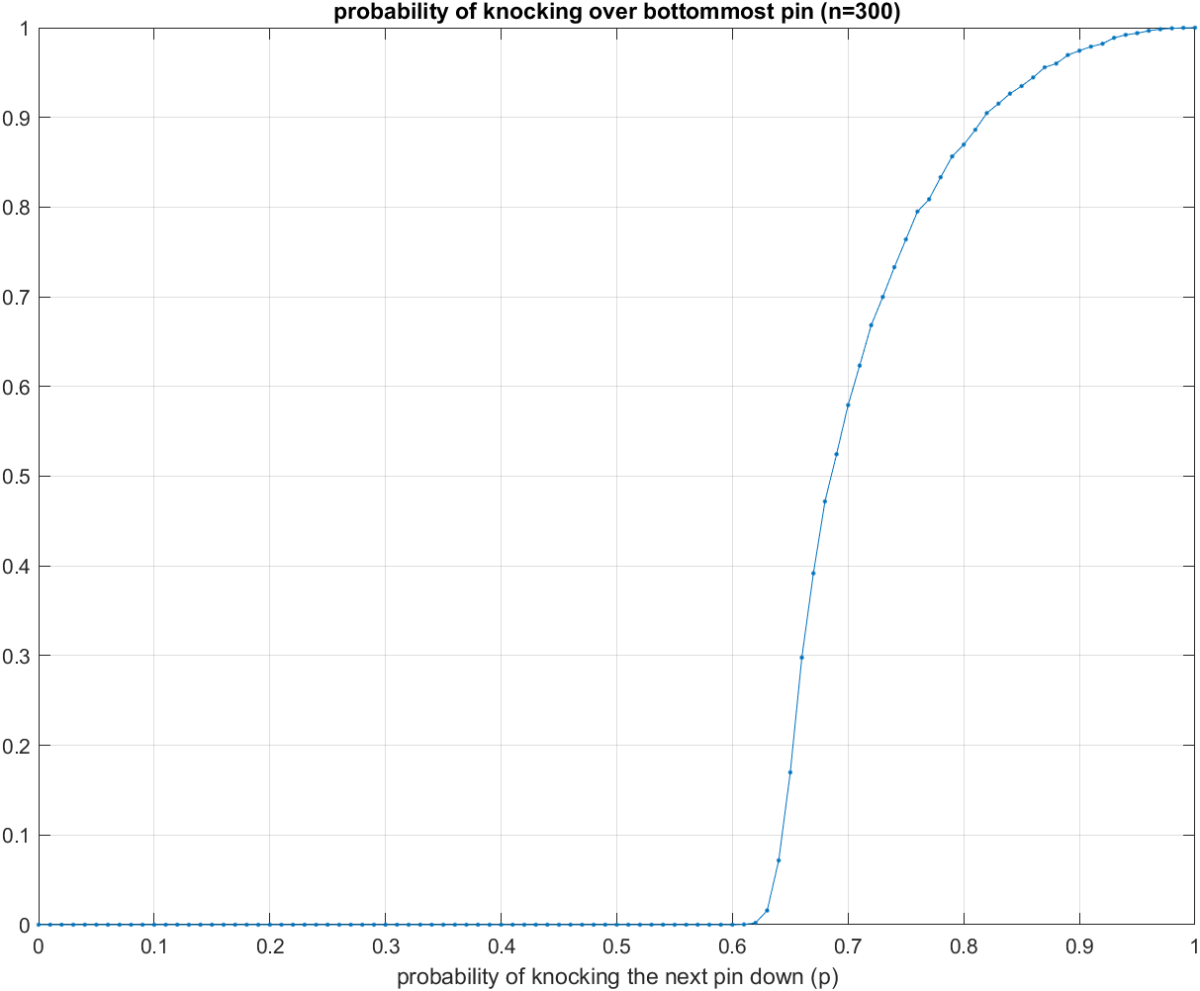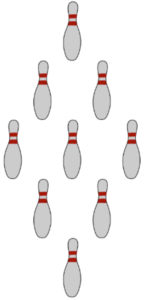# Knocking down the last bowling pinThis week’s Riddler classic is a tough one! Here is a paraphrased version of the problem.Imagine $n^2$ bowling pins arranged in a rhombus. The image to the right illustrates the case $n=3$. We knock down the topmost pin. When any pin gets knocked down, each of the (up to two) pins directly behind it has a probability $p$ of being knocked over (independently of each other). We are interested in the probability that the bottommost pin gets knocked down in the limit of large $n$.

The original problem specifically asked about $p=0.5$ and $p=0.7$.

My solution:
[Show Solution]

## 3 thoughts on “Knocking down the last bowling pin”

1.Guy D. Moore says:

This is very nice.
I also did some Monte-Carlo study of the p=0.7 case for N=2,3,…,99.
Strangely, I observe that, as N increases, the probability to succeed falls, until about N=24, and then slightly increases. The minimum is about 0.5724 and then it rises back up to about 0.5786. This surprised me and I can’t explain it.

1.Laurent says:

Yes, I noticed the same thing; couldn’t explain it either. Emily Boyajian also pointed this out in her solution. She also investigated some scenarios I didn’t consider, such as plotting the distribution of the number of pins knocked down. This might provide a clue as to what’s going on.

2.Pedro says:

This reminds me of a university project where I was simulating the percolation threshold for site percolation on a square lattice (forest fire simulation). I remember that it was possible to estimate the threshold from a power law relationship as a function of n. Do you know if it’s possible to do the same for this type of lattice?